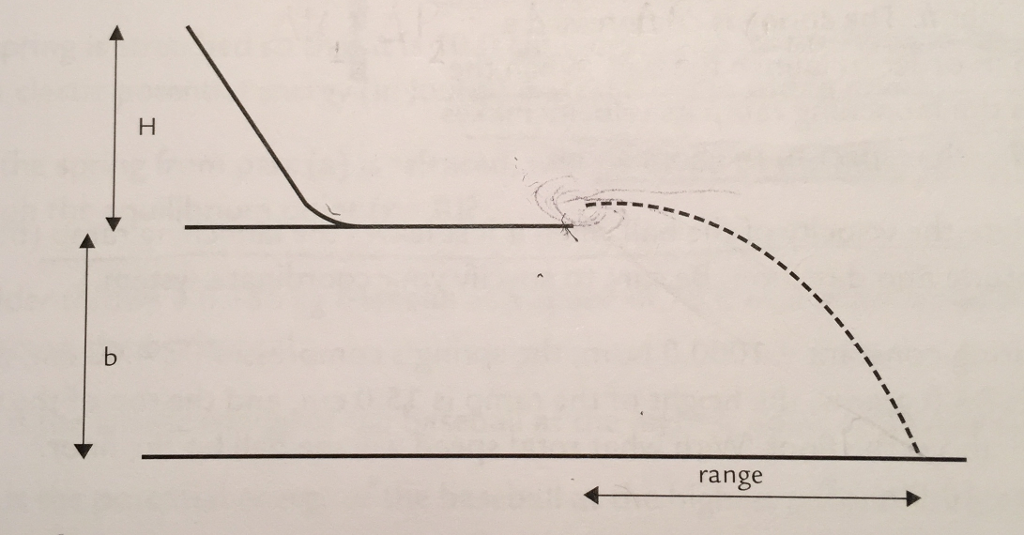# Problem: 1. Use H and b values with uncertainty to calculate the predicted range and its uncertainty in meters. Compare your predicted range to your measured range: are they consistent? Can you determine whether energy has been lost on the ramp?2. Assume that the energy lost was entirely due to friction and that the total energy of the PVC pipe is 1 meter. Use this length to compute the average force of friction. Let the values of b and H, Range measured in an experiment be:H = 64.8 ± 0.05cmb = 92.5 ± 0.05 cmRange = 120 ± 0.05cmm = 16.3g

###### FREE Expert Solution

Law of conservation of energy:

$\overline{){{\mathbf{K}}}_{{\mathbf{i}}}{\mathbf{+}}{{\mathbf{U}}}_{{\mathbf{i}}}{\mathbf{+}}{{\mathbf{W}}}_{{\mathbf{nc}}}{\mathbf{=}}{{\mathbf{K}}}_{{\mathbf{f}}}{\mathbf{+}}{{\mathbf{U}}}_{{\mathbf{f}}}}$, where Wnc is the work done by non-conservative forces such as friction.

We are required to apply the concept of uncertainty.

Rules for handling propagation of uncertainties:

Rule 1: When adding or subtracting quantities with uncertainties, we add the absolute uncertainties

Rule 2: When multiplying the quantity by a constant, the absolute uncertainty should be multiplied by the constant.

87% (196 ratings)###### Problem Details1. Use H and b values with uncertainty to calculate the predicted range and its uncertainty in meters. Compare your predicted range to your measured range: are they consistent? Can you determine whether energy has been lost on the ramp?

2. Assume that the energy lost was entirely due to friction and that the total energy of the PVC pipe is 1 meter. Use this length to compute the average force of friction.

Let the values of b and H, Range measured in an experiment be:

H = 64.8 ± 0.05cm

b = 92.5 ± 0.05 cm

Range = 120 ± 0.05cm

m = 16.3g